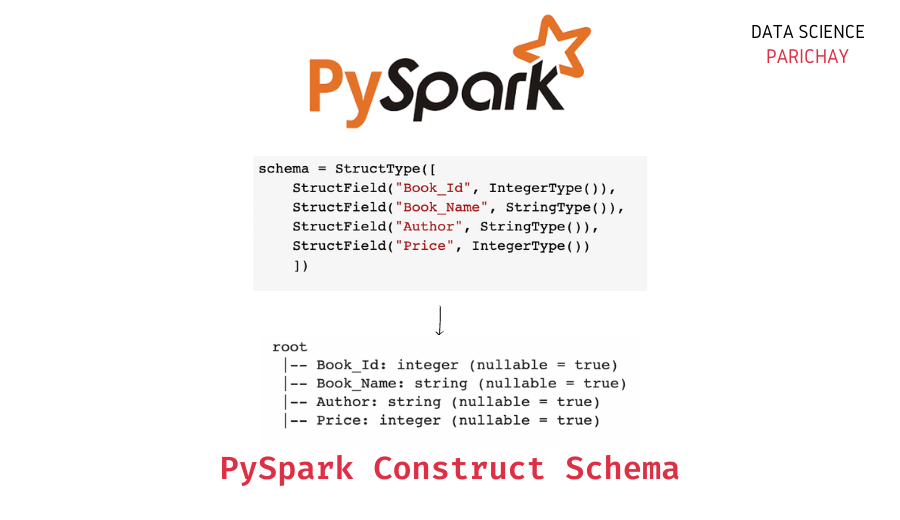# Pyspark DataFrame Schema with StructType() and StructField()

In this tutorial, we will look at how to construct schema for a Pyspark dataframe with the help of `Structype()` and `StructField()` in Pyspark.

## Pyspark Dataframe Schema

The schema for a dataframe describes the type of data present in the different columns of the dataframe. Let’s look at an example.

```#import the pyspark module
import pyspark

# import the  sparksession class  from pyspark.sql
from pyspark.sql import SparkSession

# create an app from SparkSession class
spark = SparkSession.builder.appName('datascience_parichay').getOrCreate()

# books data as list of lists
df = [[1, "PHP", "Sravan", 250],
[2, "SQL", "Chandra", 300],
[3, "Python", "Harsha", 250],
[4, "R", "Rohith", 1200],
]

# creating dataframe from books data
dataframe = spark.createDataFrame(df, ['Book_Id', 'Book_Name', 'Author', 'Price'])

# display the dataframe schema
dataframe.printSchema()```

Output:

```root
|-- Book_Id: long (nullable = true)
|-- Book_Name: string (nullable = true)
|-- Author: string (nullable = true)
|-- Price: long (nullable = true)```

Here, we created a Pyspark dataframe without explicitly specifying its schema. We then printed out the schema in tree form with the help of the `printSchema()` function.

You can see that the schema tells us about the column name and the type of data present in each column. In this case, it inferred the schema from the data itself. You can, however, specify your own schema for a dataframe.

## Construct Schema for a DataFrame

You can construct schema for a dataframe in Pyspark with the help of the `StructType()` and the `StructField()` functions. This lets you specify the type of data that you want to store in each column of the dataframe.

### StructField()

The `StructField()` function present in the `pyspark.sql.types` class lets you define the datatype for a particular column. Commonly used datatypes are `IntegerType()`, `LongType()`, `StringType()`, `FloatType()`, etc.

### StructType()

The `StructType()` function present in the `pyspark.sql.types` class lets you define the datatype for a row. That is, using this you can determine the structure of the dataframe. You can think of it as an array or list of different `StructField()`.

📚 Data Science Programs By Skill Level

Introductory

Intermediate ⭐⭐⭐

🔎 Find Data Science Programs 👨‍💻 111,889 already enrolled

Disclaimer: Data Science Parichay is reader supported. When you purchase a course through a link on this site, we may earn a small commission at no additional cost to you. Earned commissions help support this website and its team of writers.

`StructType()` can also be used to create nested columns in Pyspark dataframes.

You can use the `.schema` attribute to see the actual schema (with `StructType()` and `StructField()`) of a Pyspark dataframe. Let’s see the schema for the above dataframe.

```# dataframe schema
print(dataframe.schema)```

Output:

`StructType(List(StructField(Book_Id,LongType,true),StructField(Book_Name,StringType,true),StructField(Author,StringType,true),StructField(Price,LongType,true)))`

### Examples

Let’s look at some examples of using the above methods to create schema for a dataframe in Pyspark.

We create the same dataframe as above but this time we explicitly specify our schema.

```#import the pyspark module
import pyspark

# import the  sparksession class  from pyspark.sql
from pyspark.sql import SparkSession

# import types for building schema
from pyspark.sql.types import StructType,StructField, StringType, IntegerType

# create an app from SparkSession class
spark = SparkSession.builder.appName('datascience_parichay').getOrCreate()

# create dataframe schema
schema = StructType([
StructField("Book_Id", IntegerType()),
StructField("Book_Name", StringType()),
StructField("Author", StringType()),
StructField("Price", IntegerType())
])

# books data as list of records
# books data as list of lists
df = [[1, "PHP", "Sravan", 250],
[2, "SQL", "Chandra", 300],
[3, "Python", "Harsha", 250],
[4, "R", "Rohith", 1200],
]

# creating dataframe from schema
dataframe = spark.createDataFrame(df, schema)

# display the dataframe schema
dataframe.printSchema()```

Output:

```root
|-- Book_Id: integer (nullable = true)
|-- Book_Name: string (nullable = true)
|-- Author: string (nullable = true)
|-- Price: integer (nullable = true)```

You can see the resulting dataframe and its schema. Here the “Book_Id” and the “Price” columns are of type integer because the schema explicitly specifies them to be integer.

Let’s now use `StructType()` to create a nested column. For example, we can create a nested column for the “Author” column with two sub-columns – “First Name” and “Last Name”.

```#import the pyspark module
import pyspark

# import the  sparksession class  from pyspark.sql
from pyspark.sql import SparkSession

# import types for building schema
from pyspark.sql.types import StructType,StructField, StringType, IntegerType

# create an app from SparkSession class
spark = SparkSession.builder.appName('datascience_parichay').getOrCreate()

# create dataframe schema
schema = StructType([
StructField("Book_Id", IntegerType()),
StructField("Book_Name", StringType()),
StructField("Author", StructType([
StructField("First Name", StringType()),
StructField("Last Name", StringType())])),
StructField("Price", IntegerType())
])

# books data as list of records
df = [[1, 'PHP', ['Sravan', 'Kumar'], 250],
[2, 'SQL', ['Chandra', 'Sethi'], 300],
[3, 'Python', ['Harsha', 'Patel'], 250],
[4, 'R', ['Rohith', 'Samrat'], 1200],

# creating dataframe from schema
dataframe = spark.createDataFrame(df, schema)

# display the dataframe
dataframe.show()```

Output:

```+-------+---------+----------------+-----+
|Book_Id|Book_Name|          Author|Price|
+-------+---------+----------------+-----+
|      1|      PHP| {Sravan, Kumar}|  250|
|      2|      SQL|{Chandra, Sethi}|  300|
|      3|   Python| {Harsha, Patel}|  250|
|      4|        R|{Rohith, Samrat}| 1200|
|      5|   Hadoop| {Manasa, Gopal}|  700|
+-------+---------+----------------+-----+```

Let’s now display the schema for this dataframe.

```# display the dataframe schema
dataframe.printSchema()```

Output:

```root
|-- Book_Id: integer (nullable = true)
|-- Book_Name: string (nullable = true)
|-- Author: struct (nullable = true)
|    |-- First Name: string (nullable = true)
|    |-- Last Name: string (nullable = true)
|-- Price: integer (nullable = true)
```

The schema shows the nested column structure present in the dataframe.

You might also be interested in –

••